Courses

# Principles of Negative Voltage Feedback In Amplifiers Electrical Engineering (EE) Notes | EduRev

## Electronic Devices

Created by: Cstoppers Instructors

## Electrical Engineering (EE) : Principles of Negative Voltage Feedback In Amplifiers Electrical Engineering (EE) Notes | EduRev

The document Principles of Negative Voltage Feedback In Amplifiers Electrical Engineering (EE) Notes | EduRev is a part of the Electrical Engineering (EE) Course Electronic Devices.
All you need of Electrical Engineering (EE) at this link: Electrical Engineering (EE)

Principles of Negative Voltage Feedback In Amplifiers

A feedback amplifier has two parts viz an amplifier and a feedback circuit. The feedback circuit usually consists of resistors and returns a fraction of output energy back to the input. Fig. 1.3 shows the principles of negative voltage feedback in an amplifier. Typical values have been assumed to make the treatment more illustrative.
The output of the amplifier is 10 V. The fraction mV of this output i.e. 100 mV is fedback to the input where it is applied in series with the input signal of 101 mV. As the feedback is negative, therefore, only 1 mV appears at the input terminals of the amplifier. Referring to Fig. 1.3, we have, Gain of amplifier without feedback,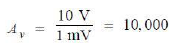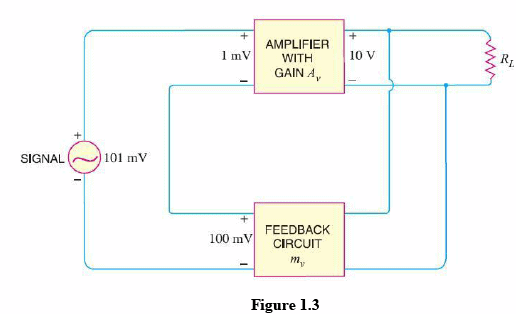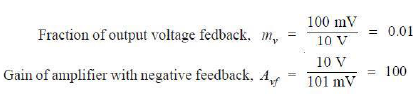The following points are worth noting :

• When negative voltage feedback is applied, the gain of the amplifier is reduced.
• Thus, the gain of above amplifier without feedback is 10,000 whereas with negative feedback, it is only 100.
• When negative voltage feedback is employed, the voltage actually applied to the amplifier is extremely  small. In this case, the signal voltage is 101 mV and the negative feedback is 100 mV so that voltage applied at the input of the amplifier is only 1 mV.
• In a negative voltage feedback circuit, the feedback fraction mV is always between 0 and 1.
• The gain with feedback is sometimes called closed- loop gain while the gain without feedback is called open-loop gain. These terms come from the fact that amplifier and feedback circuits form a loop. When the loop  is ―opened by disconnecting the feedback circuit from the input, the amplifier's gain is Av, the open- loop gain. When the loop is closed b y connecting the feedback circuit, the gain decreases to Avf, the ―closed- loop gain.

Gain of Negative Voltage Feedback Amplifier:

Consider the negative voltage feedback amplifier shown in Fig. 1.4. The gain of the amplifier without feedback is Av. Negative feedback is then applied by feeding a fraction mv of the output voltage eo back to amplifier input. Therefore, the actual input to the amplifier is the signal voltage eg minus feedback voltage mv e0 i.e.,

Actual input to amplifier = eg − mv e0

The output e0 must be equal to the input voltage (eg − mv e0) multiplied by gain Av of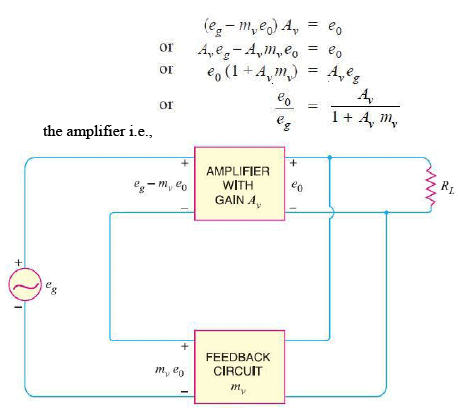But e0/eg is the voltage gain of the amplifier with feedback. Voltage gain with negative feedback is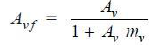It may be seen that the gain of the amplifier without feedback is Av. However, when negative voltage feedback is applied, the gain is reduced by a factor 1 + Av mv. It may be noted that negative voltage feedback does not affect the current gain of the circuit.

The following are the advantages of negative voltage feedback in amplifiers :

(i) Gain stability. An important advantage of negative voltage feedback is that the resultant gain of the amplifier can be made independent of transistor parameters or the supply voltage variations.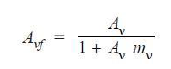for negative voltage feedback in an amplifier to be effective, the designer deliberately makes the product Av mv much greater than unity. Therefore, in the above relation, 1 can be neglected as compared to Av mv  and the expression becomes :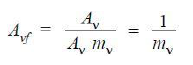It may be seen that the gain now depends only upon feedback fraction mv i.e., on the characteristics of feedback circuit. As feedback circuit is usually a voltage divider (a resistive network), therefore, it is unaffected by changes in temperature, variations in transistor parameters and frequency. Hence, the gain of the amplifier is extremely stable.

(ii) Reduces non- linear distortion. A large signal stage has non- linear distortion because its voltage gain changes at various points in the cycle. The negative voltage feedback reduces the nonlinear distortion in large signal amplifiers. It can be proved mathematically that :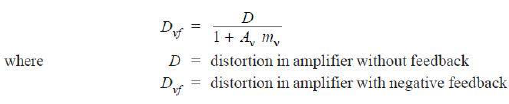It is clear that by applying negative voltage feedback to an amplifier, distortion is reduced by a factor 1 + Av mv.

(iii) Improves frequency response. As feedback is usually obtained through a resistive network, therefore, voltage gain of the amplifier is independent of signal frequency.
The result is that voltage gain of the amplifier will be substantially constant over a wide range of signal frequency. The negative voltage feedback, therefore, improves the frequency response of the amplifier.

(iv) Increases circuit stability. The output of an ordinary amplifier is easily changed due to variations in ambient temperature, frequency and signal amplitude. This changes the gain of the amplifier, resulting in distortion. However, by applying negative voltage feedback, voltage gain of the amplifier is stabilised or accurately fixed in value.

This can be easily  explained. Suppose the output of a negative voltage feedback amplifier has increased because of temperature change or due to some other reason. This means more negative feedback since feedback is being given from the output. This tends to oppose the increase in amplification and maintain it stable. The same is true should the output voltage decrease. Consequently, the circuit stability is considerably increased.

(v) Increases input impedance and decreases output impedance. The  negative voltage feedback  increases the input impedance and decreases the output impedance of amplifier. Such a change is profitable in practice as the amplifier can then serve the purpose of impedance matching.

(a) Input impedance. The increase in input impedance with negative voltage feedback can be explained by referring to Fig. 1.5. Suppose the input impedance of the amplifier is Zin without feedback and Z ′in with negative feedback. Let us further assume that input current is i1. Referring to Fig. 1.5, we have,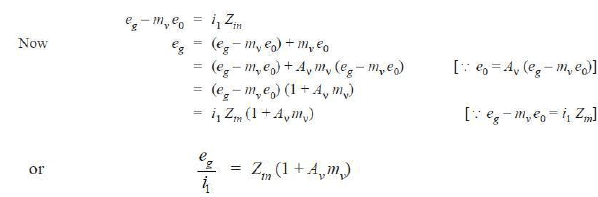But eg/i1 = Z ′in , the input impedance of the amplifier with negative voltage feedback.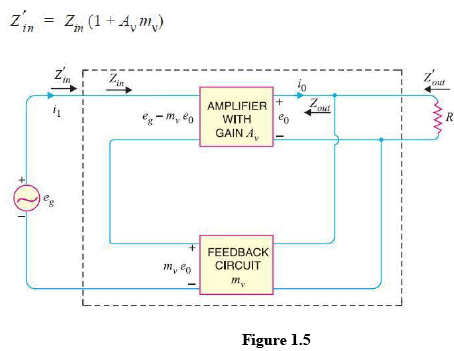It is clear that by applying negative voltage feedback, the input impedance of the amplifier is increased by a factor 1 + Aν mv. As Aν mv is much greater than unity, therefore, input impedance is increased considerably. This is an advantage, since the amplifier will now present less of a load to its source circuit.

(b) Output impedance. Following similar line, we can show that output impedance with negative voltage feedback is given by :It is clear that by applying negative feedback, the output impedance of the amplifier is decreased by a factor 1 + Aν mv . This is an added benefit of using negative voltage feedback. With lower value of output impedance, the amplifier is much better suited to drive low impedance loads.

Offer running on EduRev: Apply code STAYHOME200 to get INR 200 off on our premium plan EduRev Infinity!

,

,

,

,

,

,

,

,

,

,

,

,

,

,

,

,

,

,

,

,

,

;